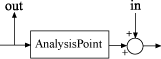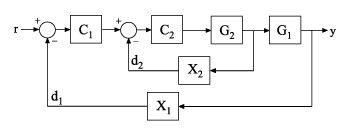getIOTransfer

Closed-loop transfer function from generalized model of control system

Description

example

H = getIOTransfer(T,in,out) returns the transfer function from specified inputs to specified outputs of a control system, computed from a closed-loop generalized model of the control system.

example

H = getIOTransfer(T,in,out,openings) returns the transfer function calculated with one or more loops open.

Examples

collapse all

Analyze responses of a control system by using getIOTransfer to compute responses between various inputs and outputs of a closed-loop model of the system.

Consider the following control system.Create a genss model of the system by specifying and connecting the numeric plant models G1 and G2, the tunable controllers C1 and C2, and the AnalysisPoint blocks X1 and X2 that mark potential loop-opening or signal injection sites.

G1 = tf(10,[1 10]);
G2 = tf([1 2],[1 0.2 10]);
C1 = tunablePID('C','pi');
C2 = tunableGain('G',1);
X1 = AnalysisPoint('X1');
X2 = AnalysisPoint('X2');
T = feedback(G1*feedback(G2*C2,X2)*C1,X1);
T.InputName = 'r';
T.OutputName = 'y';

If you tuned the free parameters of this model (for example, using the tuning command systune), you might want to analyze the tuned system performance by examining various system responses.

For example, examine the response at the output, y, to a disturbance injected at the point ${d}_{1}$.

H1 = getIOTransfer(T,'X1','y');

H1 represents the closed-loop response of the control system to a disturbance injected at the implicit input associated with the AnalysisPoint block X1, which is the location of ${d}_{1}$:H1 is a genss model that includes the tunable blocks of T. If you have tuned the free parameters of T, H1 allows you to validate the disturbance response of your tuned system. For example, you can use analysis commands such as bodeplot or stepplot to examine the responses of H1. You can also use getValue to obtain the current value of H1, in which all the tunable blocks are evaluated to their current numeric values.

Similarly, examine the response at the output to a disturbance injected at the point ${d}_{2}$.

H2 = getIOTransfer(T,'X2','y');

You can also generate a two-input, one-output model representing the response of the control system to simultaneous disturbances at both ${d}_{1}$ and ${d}_{2}$. To do so, provide getIOTransfer with a cell array that specifies the multiple input locations.

H = getIOTransfer(T,{'X1','X2'},'y');

Compute the response from r to y of the following cascaded control system, with the inner loop open, and the outer loop closed.Create a genss model of the system by specifying and connecting the numeric plant models G1 and G2, the tunable controllers C1 and C2, and the AnalysisPoint blocks X1 and X2 that mark potential loop-opening or signal injection sites.

G1 = tf(10,[1 10]);
G2 = tf([1 2],[1 0.2 10]);
C1 = tunablePID('C','pi');
C2 = tunableGain('G',1);
X1 = AnalysisPoint('X1');
X2 = AnalysisPoint('X2');
T = feedback(G1*feedback(G2*C2,X2)*C1,X1);
T.InputName = 'r';
T.OutputName = 'y';

If you tuned the free parameters of this model (for example, using the tuning command systune), you might want to analyze the tuned system performance by examining various system responses.

For example, compute the response of the system with the inner loop open, and the outer loop closed.

H = getIOTransfer(T,'r','y','X2');

By default, the loops are closed at the analysis points X1 and X2. Specifying 'X2' for the openings argument causes getIOTransfer to open the loop at X2 for the purposes of computing the requested transfer from r to y. The switch at X1 remains closed for this computation.

Input Arguments

collapse all

Model of a control system, specified as a generalized state-space model (genss).

Input to extracted transfer function, specified as a character vector or cell array of character vectors. To extract a multiple-input transfer function from the control system, use a cell array of character vectors. Each specified input must match either:

• An input of the control system model T; that is, a channel name fromT.InputName.

• An analysis point in T, corresponding to a channel of an AnalysisPoint block in T. To get the list of available analysis points in T, use getPoints(T).

When you specify an analysis point as an input in, getIOTransfer uses the input implicitly associated with the AnalysisPoint channel, arranged as follows.This input signal models a disturbance entering at the output of the switch.

If an analysis point has the same name as an input of T, then getIOTransfer uses the input of T.

Example: {'r','X1'}

Output of extracted transfer function, specified as a character vector or cell array of character vectors. To extract a multiple-output transfer function from the control system, use a cell array of character vectors. Each specified output must match either:

• An output of the control system model T; that is, a channel name from T.OutputName.

• An analysis point in T, corresponding to a channel of an AnalysisPoint block in T. To get the list of available analysis points in T, use getPoints(T).

When you specify an analysis point as an output out, getIOTransfer uses the output implicitly associated with the AnalysisPoint channel, arranged as follows.If an analysis point has the same name as an output of T, then getIOTransfer uses the output of T.

Example: {'y','X2'}

Locations for opening feedback loops for computation of the response from in to out, specified as a character vector or cell array of character vectors that identify analysis points in T. Analysis points are marked by AnalysisPoint blocks in T. To get the list of available analysis points in T, use getPoints(T).

Use openings when you want to compute the response from in to out with some loops in the control system open. For example, in a cascaded loop configuration, you can calculate the response from the system input to the system output with the inner loop open.

Output Arguments

collapse all

Closed-loop transfer function of the control system T from in to out, returned as a generalized state-space model (genss).

• If both in and out specify a single signal, then T is a SISO genss model.

• If in or out specifies multiple signals, then T is a MIMO genss model.

Tips

• You can use getIOTransfer to extract various subsystem responses, given a generalized model of the overall control system. This is useful for validating responses of a control system that you tune with tuning commands such as systune.

For example, in addition to evaluating the overall response of a tuned control system from inputs to outputs, you can use getIOTransfer to extract the transfer function from a disturbance input to a system output. Evaluate the responses of that transfer function (such as with step or bode) to confirm that the tuned system meets your disturbance rejection requirements.

• getIOTransfer is the genss equivalent to the Simulink® Control Design™ getIOTransfer (Simulink Control Design) command, which works with the slTuner and slLinearizer interfaces. Use the Simulink Control Design command when your control system is modeled in Simulink.